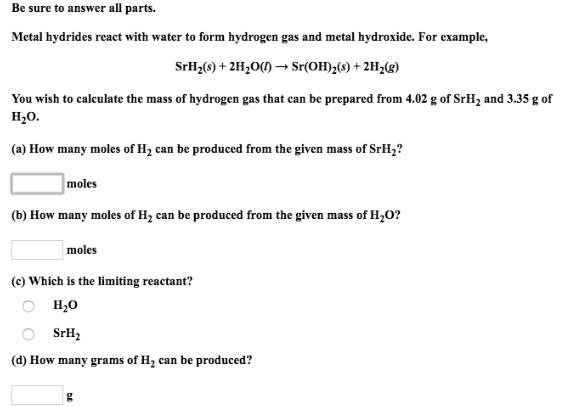# Problem: Be sure to answer all parts. Metal hydrides react with water to form hydrogen gas and metal hydroxide. For example, SrH2(s) + 2H2O(l) → Sr(OH)2(s) + 2H2(g) You wish to calculate the mass of hydrogen gas that can be prepared from 4.02 g of SrH2 and 3.35 g of H2O. (a) How many moles of H2 can be produced from the given mass of SrH2? (b) How many moles of H2 can be produced from the given mass of H2O? (c) Which is the limiting reactant?                           • H2O                           • SrH2 (d) How many grams of H2 can be produced?

###### FREE Expert Solution
80% (396 ratings)###### Problem Details

Be sure to answer all parts.

Metal hydrides react with water to form hydrogen gas and metal hydroxide. For example,

SrH2(s) + 2H2O(l) → Sr(OH)2(s) + 2H2(g)

You wish to calculate the mass of hydrogen gas that can be prepared from 4.02 g of SrH2 and 3.35 g of H2O.

(a) How many moles of H2 can be produced from the given mass of SrH2

(b) How many moles of H2 can be produced from the given mass of H2O?

(c) Which is the limiting reactant?

• H2

• SrH2

(d) How many grams of H2 can be produced?